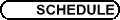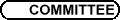SS1 - Algèbres d'opérateurs / SS1 - Operator algebras
Org: C. Anantharaman (Orléans) et/and I. Putnam (Victoria)

MOULAY BENAMEUR, Université de Metz
The index theorem in Haefliger cohomology

We shall first define the Gysin maps in Haefliger cohomology and show their compatibility with the Gysin maps in K-theory. This yields an easy computation of the topological longitudinal index map. When the Novikov-Shubin invariants of the foliation are greater than half the codimension, we prove that the Chern character of the analytical longitudinal index coincides with the index bundle. This improves a previous result of Heitsch and Lazarov.

Joint work with J. Heitsch.

JEROME CHABERT, Université Blaise Pascal, Clermont-Ferrand
Kuenneth formula and the Baum-Connes conjecture

In this joint work with Siegfried Echterhoff and Hervé Oyono-Oyono, we formalize a method for proving results on the topological K-theory of a group G-the left-hand side of the Baum-Connes conjecture for G-by reducing to certain compact subgroups of G. Using this method, we obtain that this conjecture is stable under various operations on the group G, and we investiguate the connections between the Kuenneth formula for the C*-algebra of G and the change of coefficient algebra in the Baum-Connes conjecture.

In particular, we show that the adelic group corresponding to a linear algebraic group over a finite extension of Q satisfies the conjecture for coefficient algebras with no action.

GEORGE ELLIOTT, University of Toronto, Toronto, Canada
Amenable C*-algebras

Recent results concerning the classification of (separable) amenable C*-algebras will be surveyed. A fairly clearly defined dichotomy is appearing between a class of especially well-behaved amenable C*-algebras, and the rest (typified by examples of Villadsen, and later also of Rordam and Toms).

HEATH EMERSON, University of Victoria, Canada
Asymptotic geometry of groups and the Novikov conjecture

We introduce the notion of an `asymptotic K-theory class' for a group G. Such classes, which are cohomology classes for BG, may be regarded as arising from dyanamics, or from large-scale geometry. Every asymptotic K-theory class satisfies the Novikov conjecture, i.e. is homotopy-invariant. The set of asymptotic K-classes may be gathered into a single geometric K-theory group which maps to the ordinary K-theory of BG. We give some examples and show how our framework allows one to prove that existence of a g-element for a group G is a quasi-isometry invariant of the group.

DAMIEN GABORIAU, ENS-Lyon, UMPA-UMR CNRS 5669, 46 allée d'Italie, 69364 Lyon Cedex 7
Some invariants for equivalence relations and factors

Type II1 equivalence relations R are produced for instance by probability measure preserving actions of countable groups or by holonomy pseudogroups of measured laminations. To such an R is associated its sequence of L2-Betti numbers. They are associated with pairs of von Neumann algebra/Cartan subalgebras. These numbers are just multiplied by a constant when one restricts R to a big enough Borel subset. This property enables S. Popa to produce the first example of a factor with a trivial fundamental group. I'll try to give some ideas about the subject.

THIERRY GIORDANO, Université d'Ottawa, Ottawa
Orbit equivalence of minimal actions on the Cantor set

Both in the measurable and in the Borel case, hyperfinite actions are well understood and classified up to orbit equivalence. In particular any free Borel action of Z2 is (orbit equivalent to) hyperfinite. In the case of (minimal) topological actions of Z2 on the Cantor set, the same question is still open. In this talk, I will present recent developments in the study of this problem. These developments come from a work in progress with I. Putnam (University of Victoria) and C. Skau (NTNU, Trondheim).

DAVID KERR, Universität Münster, Einsteinstr. 62, 48149  Münster, Germany
C*-dynamics and contractive approximation entropy

We develop a Banach space analogue of Voiculescu-Brown entropy and discuss its advantages and limitations as an invariant for C*-dynamical systems. This is joint work with Hanfeng Li.

MARCELO LACA, University of Victoria
Boundary quotients of Toeplitz algebras of Artin groups

I will talk about the Toeplitz C*-algebras of Artin groups of rectangular type, and the simple quotients that arise from considering a certain partial action on the boundary determined by the corresponding Artin monoid.

Joint work with J. Crisp.

SEVERINO T. MELO, Universidade de Sao Paulo, Caixa Postal 66281, 05311-970, Sao Paulo, Brazil
A K-theoretic proof of Boutet de Monvel's index theorem

Let A denote the C*-closure of the algebra of all polyhomogeneous operators of order and class zero in Boutet de Monvel's calculus on a connected compact manifold X with nonempty boundary. We derive a natural short exact sequence

 0® Ki æè C(X) öø ® Ki (A/K) ® K1-i (TX¢) ® 0,
which splits in a not necessarily natural way. Here K is the compact ideal and TX¢ is the cotangent bundle of the interior of X.

We also give a K-theoretic proof of the fact that the composition of the topological index with the mapping from K1 (A/K) to K0 (TX¢) in the above sequence gives the Fredholm-index homomorphism. That the Fredholm index for A factors through K(TX¢) was first proven by Boutet de Monvel.

This is joint work with Thomas Schick and Elmar Schrohe, based on previous joint work with Ryszard Nest and Elmar Schrohe.

JEAN RENAULT, Université d'Orléans, BP 6759, 45067 Orléans Cedex 2
Mesures de Gibbs sur les relations d'équivalence AP

Il s'agit de déterminer l'ensemble, ou au moins d'étudier l'existence et l'unicité, des mesures quasi-invariantes sur une relation d'équivalence admettant un cocycle donné comme dérivée de Radon-Nikodym. Quand la relation d'équivalence est approximativement propre, c'est-à-dire réunion d'une suite croissante de relations d'équivalence propres, on peut utiliser la caractérisation de Dobrushin, Lanford et Ruelle des mesures de Gibbs et des résultats de Goodearl sur les groupes de dimension pour établir dans certains cas l'unicité. Nous présenterons aussi une version C*-algébrique de ces résultats, dont certains ont été obtenus en collaboration avec R. Exel et A. Kumjian.